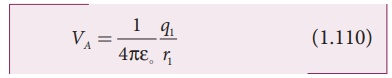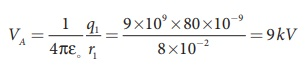Home | | Physics 12th Std | Distribution of charges in a conductor

# Distribution of charges in a conductor

The distance between the spheres is much greater than the radii of either spheres.

Distribution of charges in a conductor

Consider two conducting spheres A and B of radii r1 and r2 respectively connected to each other by a thin conducting wire as shown in the Figure 1.62. The distance between the spheres is much greater than the radii of either spheres.If a charge Q is introduced into any one of the spheres, this charge Q is redistributed into both the spheres such that the electrostatic potential is same in both the spheres. They are now uniformly charged and attain electrostatic equilibrium. Let q1 be the charge residing on the surface of sphere A and q2 is the charge residing on the surface of sphere B such that Q = q1 + q2. The charges are distributed only on the surface and there is no net charge inside the conductor.

The electrostatic potential at the surface of the sphere A is given byThe electrostatic potential at the surface of the sphere B is given byThe surface of the conductor is an equipotential. Since the spheres are connected by the conducting wire, the surfaces of both the spheres together form an equipotential surface. This implies thatLet us take the charge density on the surface of sphere A is σ1 and charge density on the surface of sphere B is σ2. This implies that q1 = 4πr12σ1 and q2 = 4πr22σ2. Substituting these values into equation (1.112), we getThus the surface charge density σ is inversely proportional to the radius of the sphere. For a smaller radius, the charge density will be larger and vice versa.

EXAMPLE 1.23

Two conducting spheres of radius r1 = 8 cm and r2 = 2 cm are separated by a distance much larger than 8 cm and are connected by a thin conducting wire as shown in the figure. A total charge of Q = +100 nC is placed on one of the spheres. After a fraction of a second, the charge Q is redistributed and both the spheres attain electrostatic equilibrium.(a) Calculate the charge and surface charge density on each sphere.

(b) Calculate the potential at the surface of each sphere.

Solution

(a) The electrostatic potential on the surface of the sphere A isThe electrostatic potential on the surface of the sphere A isSince VA = VB. We haveBut from the conservation of total charge, Q  = q1 + q2, we get q1 = Q – q2. By substituting this in the above equation,Note that the surface charge density is greater on the smaller sphere compared to the larger sphere (σ2 ≈ 4σ1) which confirms the result σ1 / σ1= r2 / r2.

The potential on both spheres is the same. So we can calculate the potential on any one of the spheres.Study Material, Lecturing Notes, Assignment, Reference, Wiki description explanation, brief detail
12th Physics : Electrostatics : Distribution of charges in a conductor |